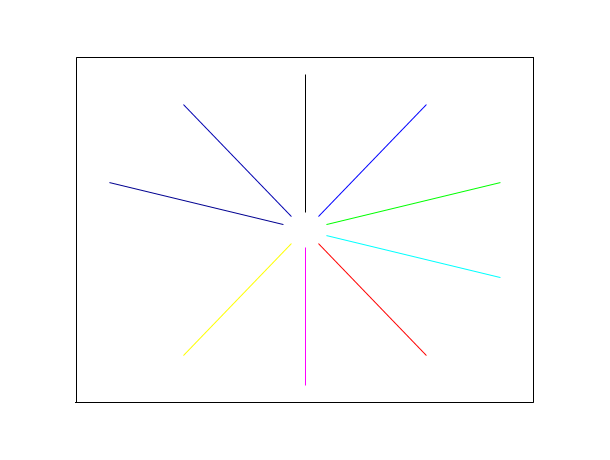Change language to:
Français - 日本語 - Português - Русский

See the recommended documentation of this function

Scilab Help >> Graphics > xsegs

# xsegs

draw unconnected segments

### Calling Sequence

```xsegs(xv, yv, [style])
xsegs(xv, yv, zv,[style])```

### Arguments

xv, yv, zv

matrices of the same size. If `zv` is not specified, a zero vector is used.

style

vector or scalar. If `style` is a positive scalar, it gives the color to use for all segments. If `style` is a negative scalar, then current color is used. If `style` is a vector, then `style(i)` gives the color to use for segment `i`.

### Description

`xsegs` draws a set of unconnected segments given by `xv`, `yv` and `zv`. If `xv`, `yv` and `zv` are matrices they are considered as vectors by concatenating their columns. The coordinates of the two points defining a segment are given by two consecutive values of `xv`, `yv` and `zv`:

`(xv(i),yv(i),zv(i))-->(xv(i+1),yv(i+1),zv(i+1))`.

For instance, using matrices of size `(2,n)`, the segments can be defined by:

```xv=[xi_1 xi_2 ...; xf_1 xf_2...]
yv=[yi_1 yi_2 ...; yf_1 yf_2...]
zv=[zi_1 zi_2 ...; zf_1 zf_2...]```

and the segments are `(xi_k,yi_k,zi_k)-->(xf_k,yf_k,zf_k)`.

### Examples

```// 2D example
x=2*%pi*(0:9)/10;
xv=[sin(x);9*sin(x)];
yv=[cos(x);9*cos(x)];
plot2d([-10,10],[-10,10],[-1,-1],"022")
xsegs(xv,yv,1:10)``````// 2D example
plot2d([-10,10],[-10,10],[-1,-1],"022")
xsegs([9, -9],[9 , -9]) // Draw the line from X(9,9) to Y(-9, -9)
xsegs([5, -2],[4 , -1]) // Draw the line from X(5,4) to Y(-2, -1)``````// 3D example
clf();
a=gca();
a.view="3d";
f=gcf();
f.color_map=rainbowcolormap(120);
alpha=2*%pi*(0:119)/40;
xv=[sin(alpha)/2;sin(alpha)/3];
yv=[cos(alpha)/2;cos(alpha)/3];
zv=[alpha/8;alpha/8];
xsegs(xv,yv,zv,1:120);